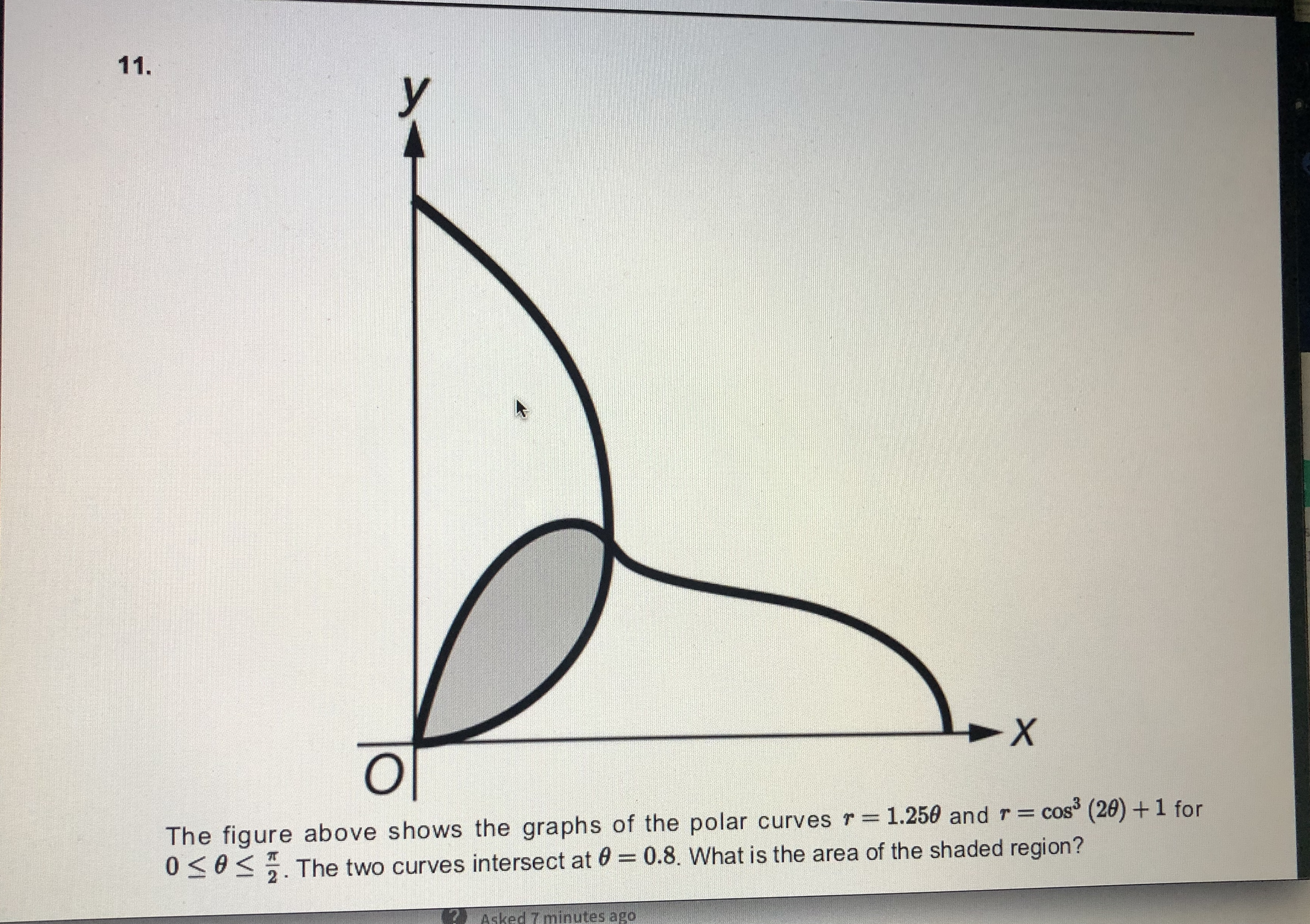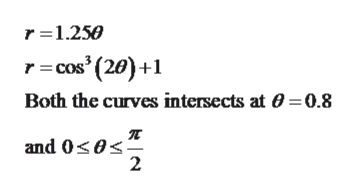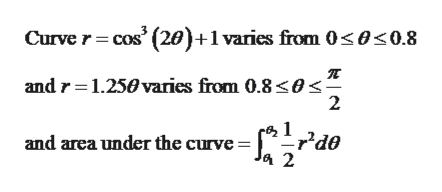# 11.The figure above shows the graphs of the polar curves r = 1.250 and r= cos (20) +1 for0305. The two curves intersect at 0 = 0.8. What is the area of the shaded region?%3DAsked 7 minutes ago

Question
23 views

Please see the picture for problem.help_outlineImage Transcriptionclose11. The figure above shows the graphs of the polar curves r = 1.250 and r= cos (20) +1 for 0305. The two curves intersect at 0 = 0.8. What is the area of the shaded region? %3D Asked 7 minutes ago fullscreen
check_circle

Step 1

Givenhelp_outlineImage Transcriptioncloser =1.250 r= cos' (20)+1 Both the curves intersects at 0 = 0.8 and 0<0<" 2 fullscreen
Step 2

Graph

Step 3

From the graph it is clear tha...help_outlineImage TranscriptioncloseCurve r= cos (20)+1 varies from 0<0<0.8 and r=1.250 varies from 0.8<0< r'de and area under the curve = Ja 2 fullscreen

### Want to see the full answer?

See Solution

#### Want to see this answer and more?

Solutions are written by subject experts who are available 24/7. Questions are typically answered within 1 hour.*

See Solution
*Response times may vary by subject and question.
Tagged in

### Math